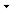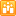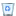# Financial## All Site Content

Displays all sites, lists, and libraries in this site.View: All Site Content### Document Libraries

There are no document libraries.

### Picture Libraries

There are no picture libraries.

### Lists

There are no lists.

### Discussion Boards

There are no discussion boards.

### Surveys

There are no surveys.

### Sites and WorkspacesDirectional Movement  The example shows how to use custom code and build in financial functions. In this case the chart displays Directional Movement indicators as a separate plot. The Directional Movement System consists of three lines: The Positive Direction Indicator (+DI) summarizes upward trend movement; The Negative Direction Indicator (-DI) summarizes downward trend movement; The Average Directional Movement Index (ADX) indicates whether the market is trending or ranging. 4 years agoMoving Averages  The example shows how to use custom code and build in financial functions. In this case the chart displays Moving Average indicators over the high, low, open or close values of the stock. A Moving Average is an indicator that shows the average value of a security's price over a period of time. A Simple, or Arithmetic, Moving Average is calculated by adding the price of the security for a number of time periods (e.g. 12 days) and then dividing this total by the number of time periods. The result is the average price of the security over the time period. Simple moving averages give equal weight to each daily price. A Weighted Moving Average is designed to put more weight on recent data and less weight on past data. A weighted moving average is calculated by multiplying each of the previous day's data by a weight (the weight is the index of the data). An Exponential Moving Average is calculated by applying a percentage of today's closing price to yesterday's moving average value. Exponential moving averages place more weight on recent prices. The Modified Moving Average is the same as the Exponential Moving Average. The only difference is in the period of the functions. For example a 14-day MMA is the same as 27-day EMA. 4 years agoPrice Indicators  The example shows how to use custom code and build in financial functions. In this case the chart displays different Price Indicators. The Median Price indicator is the midpoint of each day's price. This average price is useful when you want a simpler view of prices. The Typical Price indicator is an average of each day's price. Some investors use the Typical Price rather than the closing price when creating moving average penetration systems. The Weighted Close indicator is simply an average of each day's price. It gets its name from the fact that extra weight is given to the closing price. When plotting and back-testing moving averages, indicators, trendlines, etc, some investors like the simplicity that a line chart offers. However, line charts that only show the closing price can be misleading since they ignore the high and low price. A Weighted Close chart combines the simplicity of the line chart with the scope of a bar chart, by plotting a single point for each day that includes the high, low, and closing price. 4 years agoRange Indicators  The example shows how to use custom code and build in financial functions. In this case the chart displays different Range Indicators. Bollinger Bands are drawn at a given number of standard deviations from a moving average of a price. Since standard deviation is a measure of volatility, the bands are self-adjusting: widening during volatile markets and contracting during calmer periods. Bollinger Bands were created by John Bollinger. An Envelope is comprised of two moving averages. One moving average is shifted upward and the second moving average is shifted downward. 4 years agoRange Selection Chart  The example demonstrates how to create a combination of different Chart Areas and perform range selection (zooming) to a specific date-time range. The top chart area is displaying the detailed (zoomed) view, while the bottom chart displays the whole range (all data for the time period). When you select a From - To Date range, the top chart will be updated and the bottom chart will highlight the selected range. Chart Areas alignment on the right is achieved with custom code injection. 4 years agoTechnical Price Indicators  The example shows how to use custom code and build in financial functions. In this case the chart displays different Technical Price Indicators. - Average True Range - Chaikin's Volatility Indicator - Commodity Channel Index (CCI) - Detrended Price Oscillator - Moving Average Convergence Divergence (MACD) - Mass Index - Momentum Indicator - Momentum Division - Performance Indicator - Rate of Change Indicator - RSI - Standard Deviation - Stochastic Oscillator - TRIX - Williams %R 4 years agoVolume Indicators  The example shows how to use custom code and build in financial functions. In this case the chart displays different Volume Indicators. The Accumulation/Distribution is a momentum indicator that associates changes in price and volume. The indicator is based on the premise that the more volume that accompanies a price move, the more significant the price move. The Chaikin Oscillator is a moving average oscillator based on the Accumulation/Distribution indicator. The Ease of Movement indicator shows the relationship between volume and price change. As with Equivolume charting, this indicator shows how much volume is required to move prices. The Money Flow Index is a momentum indicator that is similar to the Relative Strength Index (RSI). It compares upward changes and downward changes of volume-weighted typical prices. This indicator can be used to identify the strength or weakness of a trend. The Negative Volume Index focuses on days where the volume decreases from the previous day. The premise being that the "smart money" takes positions on days when volume decreases. On Balance Volume is a momentum indicator that relates volume to price change. The Positive Volume Index focuses on days where the volume increased from the previous day. The premise being that the "crowd" takes positions on days when volume increases. The Price and Volume Trend is similar to On Balance Volume, in that it is a cumulative total of volume that is adjusted depending on changes in closing prices. But where OBV adds all volume on days when prices close higher and subtracts all volume on days when prices close lower, the PVT adds/subtracts only a portion of the daily volume. The amount of volume added to the PVT is determined by the amount that prices rose or fell relative to the previous day's close. 4 years ago

### Recycle BinRecycle Bin  Use this page to restore items that you have deleted from this site, or to empty deleted items. 0